##### Shhh!!! Welcome to the TransferReady worksheet library.

These stepped worksheets will help you practice single topic areas in preparation for the AQE and GL transfer tests. Bookmark this page and come back when you have identified a problem area, or just to keep up-to-speed on a certain topic as you get closer to November.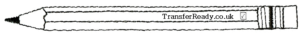###### Basic Skills are what maths teachers call “number” – adding, subtracting, multiplying, dividing and so on. In Steps 2 and 3, these basic skills are set “in context”, so candidates must begin to work out a method as well as a solution. Typically, these higher level Numeracy skills require some Literacy skills to interpret the question properly.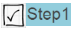Adding & Subtracting Large Numbers worksheet – basic skills practice of column addition and subtraction.Quick Multiplication worksheet – test your child’s mental multiplication skills in the 6s, 7s and 8s times tables.Midpoint of two numbers – to find the middle of two numbers, add them and divide by two.Dividing Large Numbers worksheet – with divisors of 2,3,4 and 5 only and no remainders.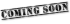Substituting numbers for letters worksheet – coming soon! Sign up to our Parents’ List to get it first.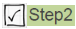Transfer Test Algebra worksheet – coming soon! Sign up to our Parents’ List to get it first.Function Machines Worksheet – An equation, such as y = 2x + 3 can be represented by the rule of a function machine. Understanding equations is essential for GCSE Maths so younger students are introduced to function machines at the end of KS2 – and they will appear on the AQE and GL transfer tests.Arithmetic Questions in Words – here are some basic arithmetic questions that are made trickier by presenting the numbers in words.Factors & Multiples – Factors and multiples form many quick, and seemingly easy, questions in the transfer tests. What they really tease out is times-tables knowledge. If you are at all shaky on your tables, you might find these challenging. Here is an entire worksheet of factors and multiples questions.Adding Decimal Numbers - Adding decimal numbers is an important step up from adding whole numbers. All the same “carry” rules apply but we need to be really careful about our placement of the decimal point. Try our "self-marking" adding worksheet to practice these vital skills.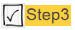Metric Unit Conversions – Accurate conversion between centimetres and metres, and other units, is an important basic skill. This worksheet covers lengths, weights and volumes.###### Nowadays, we rarely use fractions in everyday life but students are still expected to recognise and manipulate them. Pizzas are a good prop for discussions surrounding fractions: next pizza night show your child how one half equals two quarters equals four eighths. And then eat some pizza. Yum.Fractions of Amounts 1 – one over something of different amounts. Solved by just dividing by the lower number of the fraction [the denominator].Fractions of Amounts 2 – [You’ll find it just below worksheet 1.] This time divide by lower number [the denominator] and multiply by the upper number [the numerator].Equivalent Fractions 1 – Sometimes you will be asked whether two fractions have the same value (like 1/2 and 2/4) of if one fraction is more than or less than another.######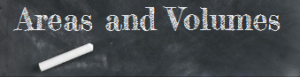Area is a measure of two-dimensional size and Volume is a measure of three-dimensional size. You are expected, in the transfer test, to be able to calculate area by counting squares but also by multiplying the sides of a rectangle. You calculate the volume of a cuboid (a three-dimensional rectangle) by multiplying three sides together.Areas of Shapes by Counting Squares - Areas of shapes is popular on the transfer tests. The most basic of these can be solved by counting 1cm² grids like in this worksheet so make sure that your child can answer these as a starting point.Areas of Shapes by Multiplying the Sides - Finding areas of squares, rectangles and triangles by multiplication.Perimeters of simple shapes - Worksheet to calculate the perimeters of some simple shapes including triangles and hexagons.Perimeters of Compound Shapes - Compound shapes are complicated 2-d shapes made up from other 2-d simple shapes - like two rectangles stuck together for example. In these questions, you will be given some (but usually not all) of the side lengths so you must work out all the missing sides and then add them together to get the perimeter.Volumes of 3-D Shapes - Volumes of cubes and cuboids is popular on the transfer tests. Calculate these by multiplying l x b x h.Volumes in real life - Real life situations involving volumes can be challenging to pupils who find it difficult to picture the question in their mind's eye. These questions, however, often only involve some simple division or multiplication and a bit of common sense.###### Statistics in the transfer test is limited to the use of some averages (AQE is only mean and range but PPTC(GL) also includes median and mode) and some statistical charts (like bar charts, pictographs and so on).Pictograms / Pictographs - A pictogram (or pictograph) is a chart that uses pictures to represent data.Four types of Average - Introductory worksheet on the four types of Average used in KS2 Maths.Applied averages worksheet - Applied problems involving mean, mode, median and range for the GL test (also useful for AQE but remember that this only examines mean and range).###### Time questions on the transfer test are often simple adding or subtracting problems, but made more challenging by being set in the context of time or date. The challenge comes from the fact that our time system is not in the slightest bit decimal – we have 60 seconds in 1 minute, 24 hours in one day, 7 days in one week and varying amounts of days in any given month.Adding times - Have you ever thought about how non-decimal our system of time is? This worksheet will help you brush up on adding times, remembering to carry when you get to 60!Subtracting times - Subtraction is particularly tricky when it comes to time calculations because your borrows are not what you are used to doing.Time Calculations set in context - Time calculations framed in the context of real-life situations.Simple Day & Date Calculations - What day is it 4 days after Tuesday? Try this worksheet of simple day and date questions.Date Range Calculations - How many days are there between the 1st of January and 4th of May? Try this tricky worksheet of date range questions.More Time & Date worksheets coming soon! Sign up to our Parents' List to get them first.###### A “sequence” in maths is a list of numbers or objects in a special order. For example, {3, 5, 7, 9, …} is a sequence starting at 3 and increasing by 2 each time. The dots (English teachers call that an ellipsis, maths teachers say dot-dot-dot) after the 9 means that the sequence continues forever. Patterns are more or less the same.Number Sequences 1 - A number sequence is generated when a certain number is repeatedly added or subtracted from a starting number. For example, start with 3 and add 5 each time.More Sequences worksheets coming soon! Sign up to our Parents' List to get them first.Visual Patterns - To answer these questions, do not try to draw the required pattern out. The key is to answering these is to spot how many matches are added each time and then continue the pattern that way.###### Geometry is one of those old-fashioned maths words, like algebra and calculus, which are a bit off-putting for many people. The word geometry  comes from ancient Greek, meaning the measurement (-metry) of land (Geo-). The Greek pharaohs used maths to calculate how much tax their farmers would pay based on how large their fields where, which required the measurement of two-dimensional space. Today, Geometry is the branch of maths involving shape and space.Identifying Quadrilaterals - Can you name all the common four sided shapes?Line Symmetry 1 & 2 - Two worksheets on line symmetry.More Geometry worksheets coming soon! Sign up to our Parents' List to get them first.Rotational Symmetry 1 - [PPTC(GL) Only] Worksheet on order of rotational symmetry.

###### Don’t forget to check our Spelling of the Day every day;Apostrophes in Contractions - A contraction is a word or phrase that has been shortened by dropping one or more letters and replacing the letters with an apostrophe. In the test, you need to be able to recognise common contractions and replace the missing letters.Punctuation worksheet - Here are a few questions similar to the common punctuation questions that appear on the AQE or GL transfer tests.Homophones worksheet - Homophones are sets of words that sound the same but have different spellings and meanings.L-sound Word Endings Worksheet - Correct L-sound word endings are easy to muddel muddle up. Read our advice and try this worksheet dealing with -le -el and -al words.-ible or -able word Endings Worksheet - Coming soon.y-Word Plural Endings Worksheet - The plural of city is cities, the plural of boy is boys. Correctly spelling these plurals involves one surprisingly simple rule.More literacy worksheets coming soon! Sign up to our Parents' List to get them first.Verbal Reasoning: Insert a Letter - With this type of question you are given two incomplete words. The last letter of the first word and the first letter of the second word is missing. The student is required to find the one letter that completes the first word and begins the second word. This is really a vocabulary challenge. Solving these involves running through the words that you know (and can spell) and determining what fits.Verbal Reasoning: Find the Two Odd-Words - Coming Soon! In these questions you are given five words. Three of these words are related to one other in some way. You are required to identify the two words that are NOT related to the other three.Verbal Reasoning: Alphabet-based Codes and Ciphers - Coming Soon! Ciphers are secret codes where each letter of a word is changed by a rule. For example, TEST is coded as UFTU when each letter is replaced by the letter immediately following it in the Alphabet.Verbal Reasoning: Synonyms - Coming Soon! In these questions you are given two groups of words. You are required to identify two words, one from each group, which have a similar meaning. For example; Group1: {Red | Tired | Car} and Group2: {Exhausted | Five | Hotel}. ANS: Tired & Exhausted.Verbal Reasoning: Identify the Word that is Hidden in a Sentence - Coming Soon! With this type of question you are given a sentence in which a four letter word is hidden between two existing words. The letters at the end of one word and the letters at the beginning of the next word make up a new four letter word. You are required to locate the pair of words that contain the hidden word.Verbal Reasoning: Identify Words with Missing Letters - Coming Soon! In these question, the capitalised word has had three OR four consecutive letters taken out. These 3 or 4 letters will themselves make one correctly spelt word without changing the order of the letters. Find the missing word. Sound STRAIGHT---WARD? FOR sure.Numerical Reasoning: Calculations with Algebra - Coming Soon!Verbal Reasoning: Words with Opposite Meanings - Coming Soon! Again, two groups of words but one word from the first group and one from the second have the opposite meaning. Identify the pair of words.Numerical Reasoning: Find the Number which completes the Calculation - Coming Soon!Verbal Reasoning: Move Letters to make New Words - Coming Soon! In these questions, you are given two words. Choose one letter that can be moved from the word on the left to the word on the right, making two new words. You cannot rearrange any letters, but the letter that you move can fit anywhere in the second word.Numerical Reasoning: Number Series & Sequences - Coming Soon!Verbal Reasoning: Letter Series and Sequences - Coming Soon!Verbal Reasoning: Complete the Sentence by Choosing the Best Word - Coming Soon! In these questions you must chose two words, one from each group in brackets that best complete the sentence.Verbal Reasoning: Word Codes - Coming Soon!Verbal Reasoning: Complete the Pair of Words - Coming Soon!Verbal Reasoning: Forming New Words - Coming Soon! In these questions, find the two words, one from each group, that together make a new, real word. [hint: the word from the first group always forms the start of the new word]Verbal Reasoning: Words with Similar Meanings - Coming Soon! In these questions you are presented with two pairs of words. The words in each pair are related to one another. Then you must choose the word from the 5 possible answers which goes equally well with either of the pair of words.Verbal Reasoning: Analogies - These are the classic 11+ Verbal Reasoning questions from years ago. In these questions you are given most of an analogy. For example, SMELL is to NOSE, as SOUND is to ... and you must choose the correct answer from a list of options.Verbal Reasoning: Pick Two Statements - Coming Soon! In these questions, you must pick two statements from those given which together combine to prove the fact, also given.More Reasoning worksheets coming soon! Sign up to our Parents' List to get them first.